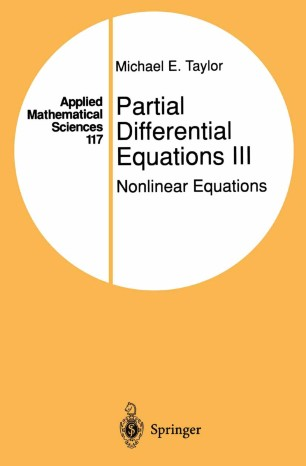# Partial Differential Equations III

## Nonlinear Equations

• Michael E. TaylorBook

Part of the Applied Mathematical Sciences book series (AMS, volume 117)

1. Front Matter
Pages i-xxi
2. Michael E. Taylor
Pages 1-88
3. Michael E. Taylor
Pages 89-270
4. Michael E. Taylor
Pages 271-358
5. Michael E. Taylor
Pages 359-465
6. Michael E. Taylor
Pages 466-523
7. Michael E. Taylor
Pages 524-605
8. Back Matter
Pages 607-611

### Introduction

Partial differential equations is a many-faceted subject. Created to describe the mechanical behavior of objects such as vibrating strings and blowing winds, it has developed into a body of material that interacts with many branches of math­ ematics, such as differential geometry, complex analysis, and harmonic analysis, as weIl as a ubiquitous factor in the description and elucidatiön of problems in mathematical physics. This work is intended to provide a course of study of some of the major aspects ofPDE.1t is addressed to readers with a background in the basic introductory grad­ uate mathematics courses in American universities: elementary real and complex analysis, differential geometry, and measure theory. Chapter 1 provides background material on the theory of ordinary differential equations (ODE). This includes both very basic material-on topics such as the existence and uniqueness of solutions to ODE and explicit solutions to equations with constant coefficients and relations to linear algebra-and more sophisticated resuIts-on ftows generated by vector fields, connections with differential geom­ etry, the calculus of differential forms, stationary action principles in mechanics, and their relation to Hamiltonian systems. We discuss equations of relativistic motion as weIl as equations of classical Newtonian mechanics. There are also applications to topological resuIts, such as degree theory, the Brouwer fixed-point theorem, and the Jordan-Brouwer separation theorem. In this chapter we also treat scalar first-order PDE, via Hamilton-Jacobi theory.

### Keywords

Gaussian curvature Minimal surface Sobolev space calculus curvature differential equation differential geometry function space hyperbolic equation mathematical physics minimum operator theory partial differential equation

#### Authors and affiliations

• Michael E. Taylor
• 1
1. 1.Department of MathematicsUniversity of North CarolinaChapel HillUSA

### Bibliographic information

• DOI https://doi.org/10.1007/978-1-4757-4190-2
• Copyright Information Springer-Verlag New York 1996
• Publisher Name Springer, New York, NY
• eBook Packages
• Print ISBN 978-1-4757-4192-6
• Online ISBN 978-1-4757-4190-2
• Series Print ISSN 0066-5452
• Buy this book on publisher's site